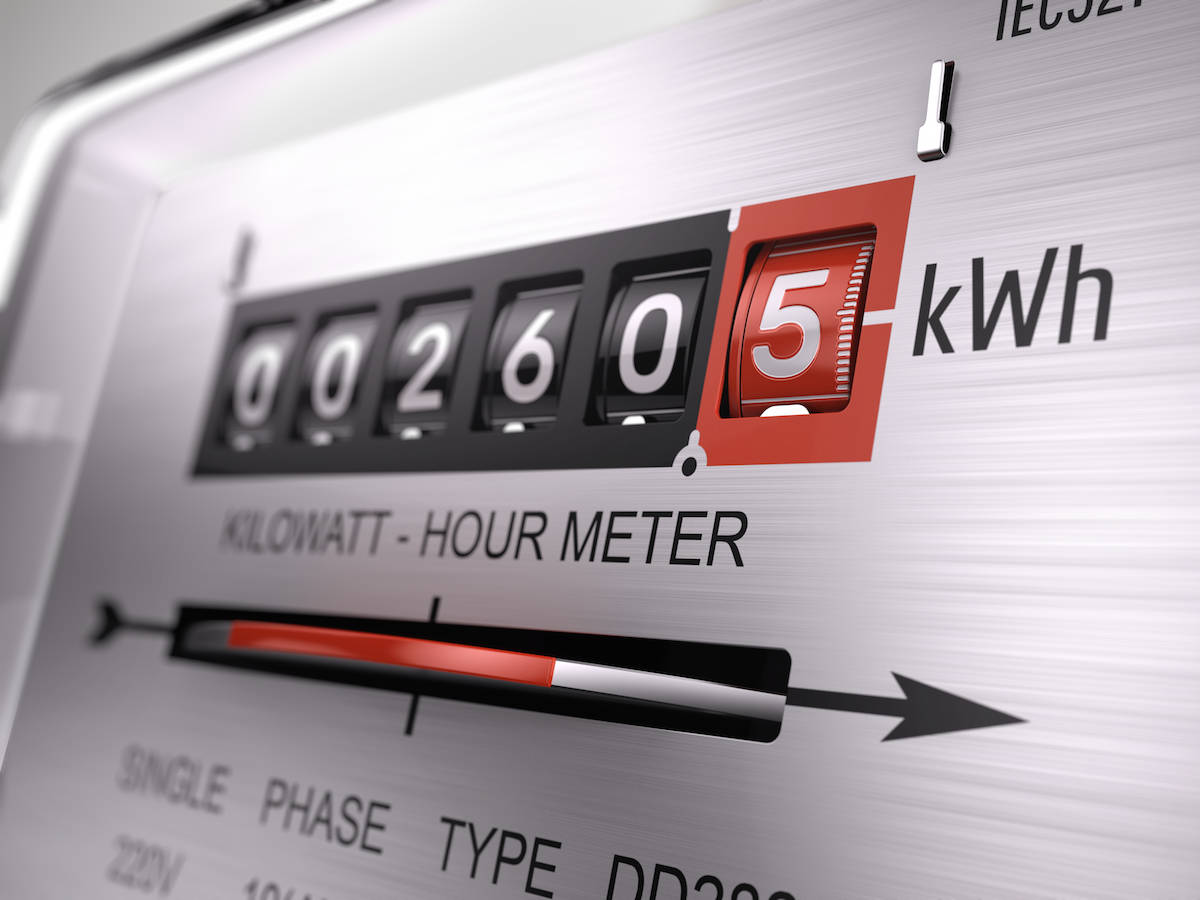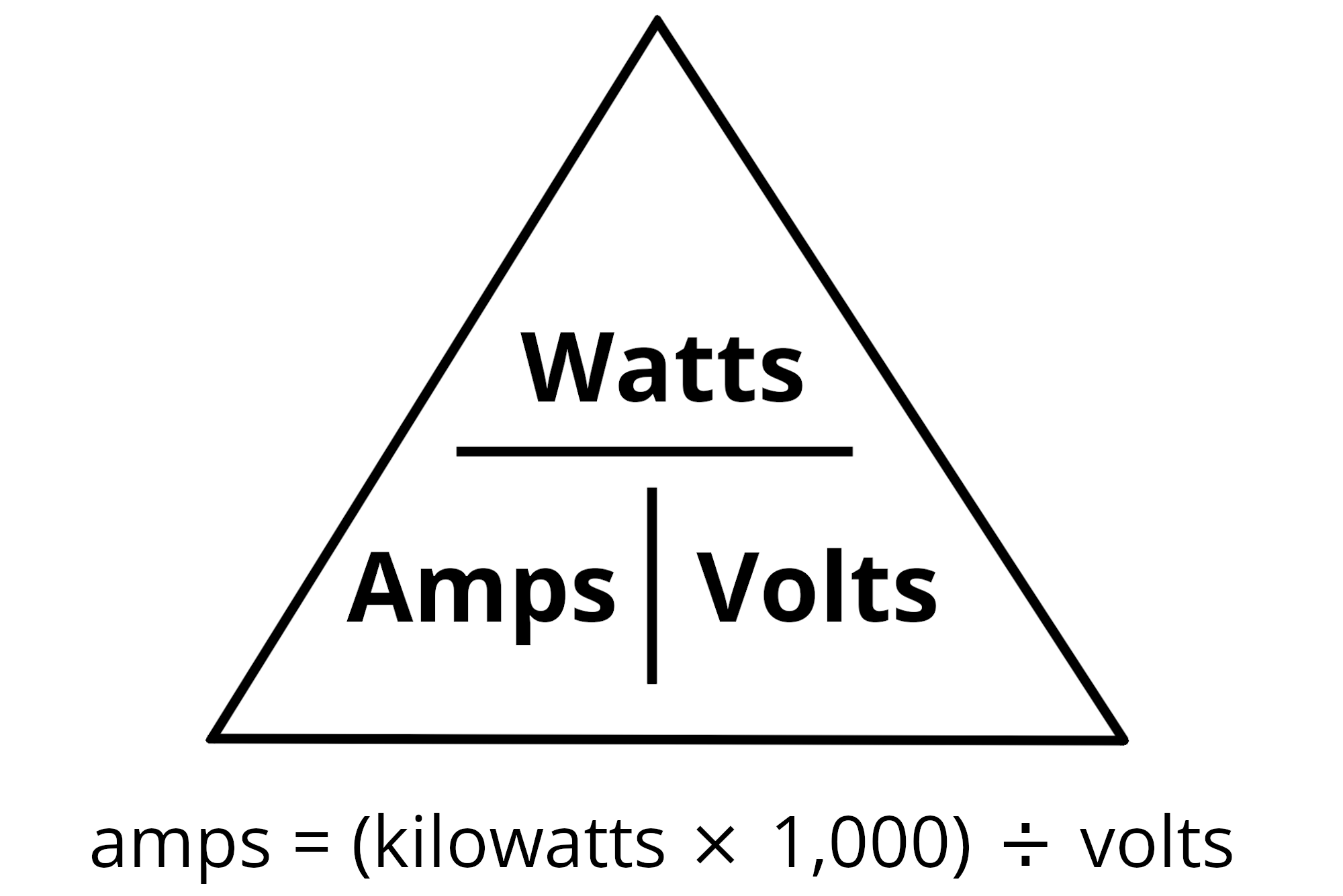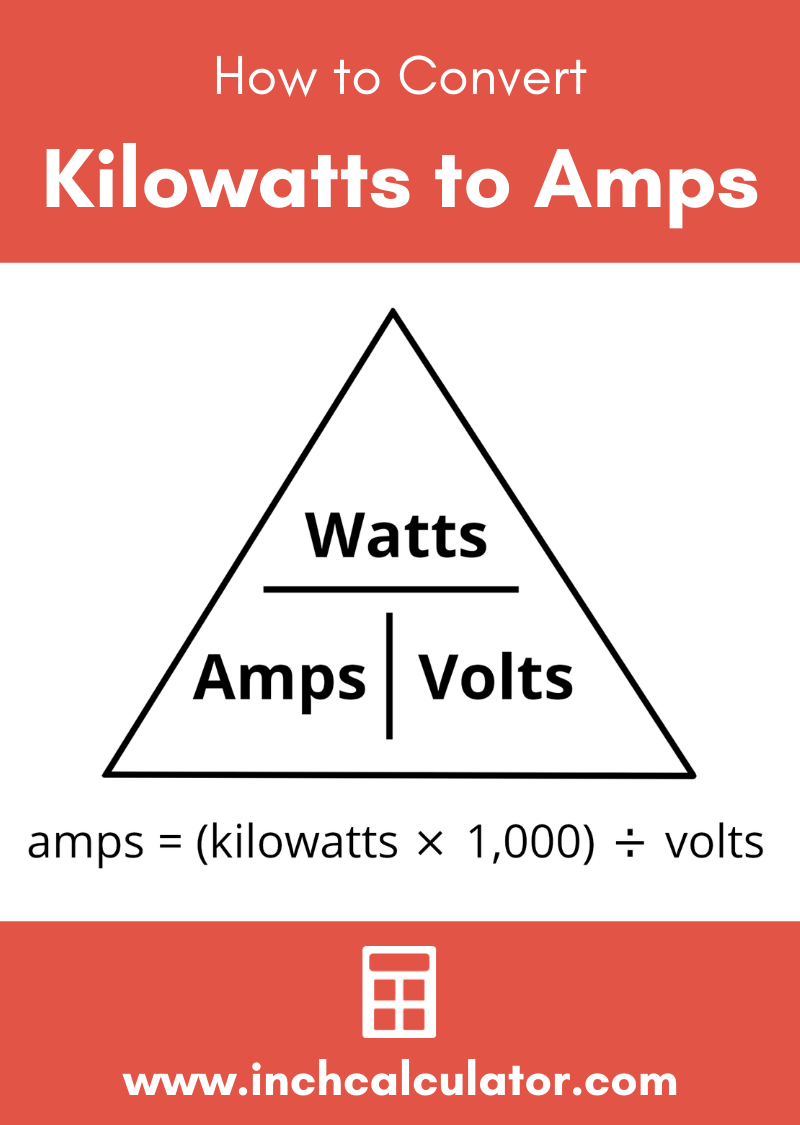# Kilowatts (kW) to Amps Electrical Conversion Calculator

amps

milliamps

## How to Convert Kilowatts to Amps

For a single phase AC circuit the formula to convert kilowatts(kW) to amps is:

amps = (kW × 1,000) ÷ volts

It is possible to find the amperage from kilowatts if you know the voltage of the circuit using Watt’s law. Watt’s Law states that current = power ÷ voltage. In Watt’s Law, power is measured in watts and voltage is measured in volts. The formula will solve for current in amps.First start by converting kilowatts to watts, which can be done by multiplying the power in kW by 1,000 to get the number of watts.

Finally, apply the Watt’s Law formula and divide the number of watts by the voltage to find the amps.For example, find the current of a circuit with 1kW of power at 120 volts.

amps = (kW × 1,000) ÷ volts
amps = (1 × 1,000) ÷ 120
amps = 1,000 ÷ 120
amps = 8.33A

### Convert Kilowatts to Amps Using a Power Factor

Equipment is often not 100% efficient with power usage and this must be factored in to find the number of amps available. For example, most generators are 80% efficient. The efficiency of the device can be converted to a power factor by converting the percentage to a decimal, this is the power factor.

To find the power factor of your circuit, try our power factor calculator.

The formula to find amps using the power factor is:

amps = (kW × 1,000) ÷ (PF × volts)

For example, find the current of a 5kW generator with an efficiency of 80% at 120 volts.

amps = (kW × 1,000) ÷ (PF × volts)
amps = (5 × 1,000) ÷ (.8 × 120)
amps = 5,000 ÷ 96
amps = 52.1A

### How to Find the Current of a Three-Phase AC Circuit

The formula to find amps for a three-phase AC circuit is a little different from the formula for a single phase circuit:

amps = (kW × 1,000) ÷ (√3 × PF × volts)

For example, find the current of a 25kW three-phase generator with an efficiency of 80% at 240 volts.

amps = (kW × 1,000) ÷ (√3 × PF × volts)
amps = (25 × 1,000) ÷ (1.73 × .8 × 240
amps = 75.18A

To convert watts to amps use our watts to amps conversion calculator.

## Generator Current Ratings (Three-Phase AC)

Generator current ratings based on kilowatt output at 120, 208, 240, 277, and 480 volts three-phase AC with a power-factor of .8
Power Current at 120V Current at 208V Current at 240V Current at 277V Current at 480V
1 kW 6.014 A 3.47 A 3.007 A 2.605 A 1.504 A
2 kW 12.028 A 6.939 A 6.014 A 5.211 A 3.007 A
3 kW 18.042 A 10.409 A 9.021 A 7.816 A 4.511 A
4 kW 24.056 A 13.879 A 12.028 A 10.421 A 6.014 A
5 kW 30.07 A 17.348 A 15.035 A 13.027 A 7.518 A
6 kW 36.084 A 20.818 A 18.042 A 15.632 A 9.021 A
7 kW 42.098 A 24.288 A 21.049 A 18.238 A 10.525 A
8 kW 48.113 A 27.757 A 24.056 A 20.843 A 12.028 A
9 kW 54.127 A 31.227 A 27.063 A 23.448 A 13.532 A
10 kW 60.141 A 34.697 A 30.07 A 26.054 A 15.035 A
15 kW 90.211 A 52.045 A 45.105 A 39.081 A 22.553 A
20 kW 120.28 A 69.393 A 60.141 A 52.107 A 30.07 A
25 kW 150.35 A 86.741 A 75.176 A 65.134 A 37.588 A
30 kW 180.42 A 104.09 A 90.211 A 78.161 A 45.105 A
35 kW 210.49 A 121.44 A 105.25 A 91.188 A 52.623 A
40 kW 240.56 A 138.79 A 120.28 A 104.21 A 60.141 A
45 kW 270.63 A 156.13 A 135.32 A 117.24 A 67.658 A
50 kW 300.7 A 173.48 A 150.35 A 130.27 A 75.176 A
55 kW 330.77 A 190.83 A 165.39 A 143.3 A 82.693 A
60 kW 360.84 A 208.18 A 180.42 A 156.32 A 90.211 A
65 kW 390.91 A 225.53 A 195.46 A 169.35 A 97.729 A
70 kW 420.98 A 242.88 A 210.49 A 182.38 A 105.25 A
75 kW 451.05 A 260.22 A 225.53 A 195.4 A 112.76 A
80 kW 481.13 A 277.57 A 240.56 A 208.43 A 120.28 A
85 kW 511.2 A 294.92 A 255.6 A 221.46 A 127.8 A
90 kW 541.27 A 312.27 A 270.63 A 234.48 A 135.32 A
95 kW 571.34 A 329.62 A 285.67 A 247.51 A 142.83 A
100 kW 601.41 A 346.97 A 300.7 A 260.54 A 150.35 A
125 kW 751.76 A 433.71 A 375.88 A 325.67 A 187.94 A
150 kW 902.11 A 520.45 A 451.05 A 390.81 A 225.53 A
175 kW 1,052.5 A 607.19 A 526.23 A 455.94 A 263.12 A
200 kW 1,202.8 A 693.93 A 601.41 A 521.07 A 300.7 A
225 kW 1,353.2 A 780.67 A 676.58 A 586.21 A 338.29 A
250 kW 1,503.5 A 867.41 A 751.76 A 651.34 A 375.88 A
275 kW 1,653.9 A 954.15 A 826.93 A 716.48 A 413.47 A
300 kW 1,804.2 A 1,040.9 A 902.11 A 781.61 A 451.05 A
325 kW 1,954.6 A 1,127.6 A 977.29 A 846.75 A 488.64 A
350 kW 2,104.9 A 1,214.4 A 1,052.5 A 911.88 A 526.23 A
375 kW 2,255.3 A 1,301.1 A 1,127.6 A 977.01 A 563.82 A
400 kW 2,405.6 A 1,387.9 A 1,202.8 A 1,042.1 A 601.41 A
425 kW 2,556 A 1,474.6 A 1,278 A 1,107.3 A 638.99 A
450 kW 2,706.3 A 1,561.3 A 1,353.2 A 1,172.4 A 676.58 A
475 kW 2,856.7 A 1,648.1 A 1,428.3 A 1,237.6 A 714.17 A
500 kW 3,007 A 1,734.8 A 1,503.5 A 1,302.7 A 751.76 A
525 kW 3,157.4 A 1,821.6 A 1,578.7 A 1,367.8 A 789.35 A
550 kW 3,307.7 A 1,908.3 A 1,653.9 A 1,433 A 826.93 A
575 kW 3,458.1 A 1,995.1 A 1,729 A 1,498.1 A 864.52 A
600 kW 3,608.4 A 2,081.8 A 1,804.2 A 1,563.2 A 902.11 A
625 kW 3,758.8 A 2,168.5 A 1,879.4 A 1,628.4 A 939.7 A
650 kW 3,909.1 A 2,255.3 A 1,954.6 A 1,693.5 A 977.29 A
675 kW 4,059.5 A 2,342 A 2,029.7 A 1,758.6 A 1,014.9 A
700 kW 4,209.8 A 2,428.8 A 2,104.9 A 1,823.8 A 1,052.5 A
725 kW 4,360.2 A 2,515.5 A 2,180.1 A 1,888.9 A 1,090 A
750 kW 4,510.5 A 2,602.2 A 2,255.3 A 1,954 A 1,127.6 A
775 kW 4,660.9 A 2,689 A 2,330.5 A 2,019.2 A 1,165.2 A
800 kW 4,811.3 A 2,775.7 A 2,405.6 A 2,084.3 A 1,202.8 A
825 kW 4,961.6 A 2,862.5 A 2,480.8 A 2,149.4 A 1,240.4 A
850 kW 5,112 A 2,949.2 A 2,556 A 2,214.6 A 1,278 A
875 kW 5,262.3 A 3,035.9 A 2,631.2 A 2,279.7 A 1,315.6 A
900 kW 5,412.7 A 3,122.7 A 2,706.3 A 2,344.8 A 1,353.2 A
925 kW 5,563 A 3,209.4 A 2,781.5 A 2,410 A 1,390.8 A
950 kW 5,713.4 A 3,296.2 A 2,856.7 A 2,475.1 A 1,428.3 A
975 kW 5,863.7 A 3,382.9 A 2,931.9 A 2,540.2 A 1,465.9 A
1000 kW 6,014.1 A 3,469.7 A 3,007 A 2,605.4 A 1,503.5 A

## Generator Current Ratings (Single Phase AC)

Generator current ratings based on kilowatt output at 120 and 240 volts single phase AC with a power factor of .8
Power Current at 120V Current at 240V
1 kW 10.417 A 5.208 A
2 kW 20.833 A 10.417 A
3 kW 31.25 A 15.625 A
4 kW 41.667 A 20.833 A
5 kW 52.083 A 26.042 A
6 kW 62.5 A 31.25 A
7 kW 72.917 A 36.458 A
8 kW 83.333 A 41.667 A
9 kW 93.75 A 46.875 A
10 kW 104.17 A 52.083 A
15 kW 156.25 A 78.125 A
20 kW 208.33 A 104.17 A
25 kW 260.42 A 130.21 A
30 kW 312.5 A 156.25 A
35 kW 364.58 A 182.29 A
40 kW 416.67 A 208.33 A
45 kW 468.75 A 234.38 A
50 kW 520.83 A 260.42 A
55 kW 572.92 A 286.46 A
60 kW 625 A 312.5 A
65 kW 677.08 A 338.54 A
70 kW 729.17 A 364.58 A
75 kW 781.25 A 390.63 A
80 kW 833.33 A 416.67 A
85 kW 885.42 A 442.71 A
90 kW 937.5 A 468.75 A
95 kW 989.58 A 494.79 A
100 kW 1,041.7 A 520.83 A
125 kW 1,302.1 A 651.04 A
150 kW 1,562.5 A 781.25 A
175 kW 1,822.9 A 911.46 A
200 kW 2,083.3 A 1,041.7 A
225 kW 2,343.8 A 1,171.9 A
250 kW 2,604.2 A 1,302.1 A
275 kW 2,864.6 A 1,432.3 A
300 kW 3,125 A 1,562.5 A
325 kW 3,385.4 A 1,692.7 A
350 kW 3,645.8 A 1,822.9 A
375 kW 3,906.3 A 1,953.1 A
400 kW 4,166.7 A 2,083.3 A
425 kW 4,427.1 A 2,213.5 A
450 kW 4,687.5 A 2,343.8 A
475 kW 4,947.9 A 2,474 A
500 kW 5,208.3 A 2,604.2 A
525 kW 5,468.8 A 2,734.4 A
550 kW 5,729.2 A 2,864.6 A
575 kW 5,989.6 A 2,994.8 A
600 kW 6,250 A 3,125 A
625 kW 6,510.4 A 3,255.2 A
650 kW 6,770.8 A 3,385.4 A
675 kW 7,031.3 A 3,515.6 A
700 kW 7,291.7 A 3,645.8 A
725 kW 7,552.1 A 3,776 A
750 kW 7,812.5 A 3,906.3 A
775 kW 8,072.9 A 4,036.5 A
800 kW 8,333.3 A 4,166.7 A
825 kW 8,593.8 A 4,296.9 A
850 kW 8,854.2 A 4,427.1 A
875 kW 9,114.6 A 4,557.3 A
900 kW 9,375 A 4,687.5 A
925 kW 9,635.4 A 4,817.7 A
950 kW 9,895.8 A 4,947.9 A
975 kW 10,156 A 5,078.1 A
1000 kW 10,417 A 5,208.3 A